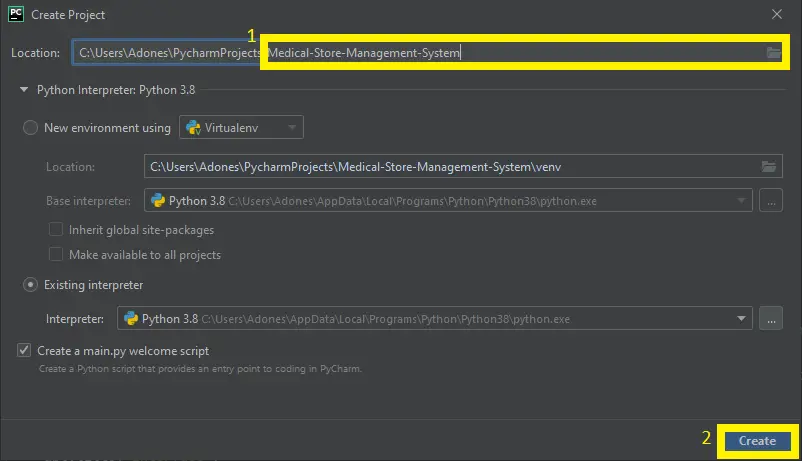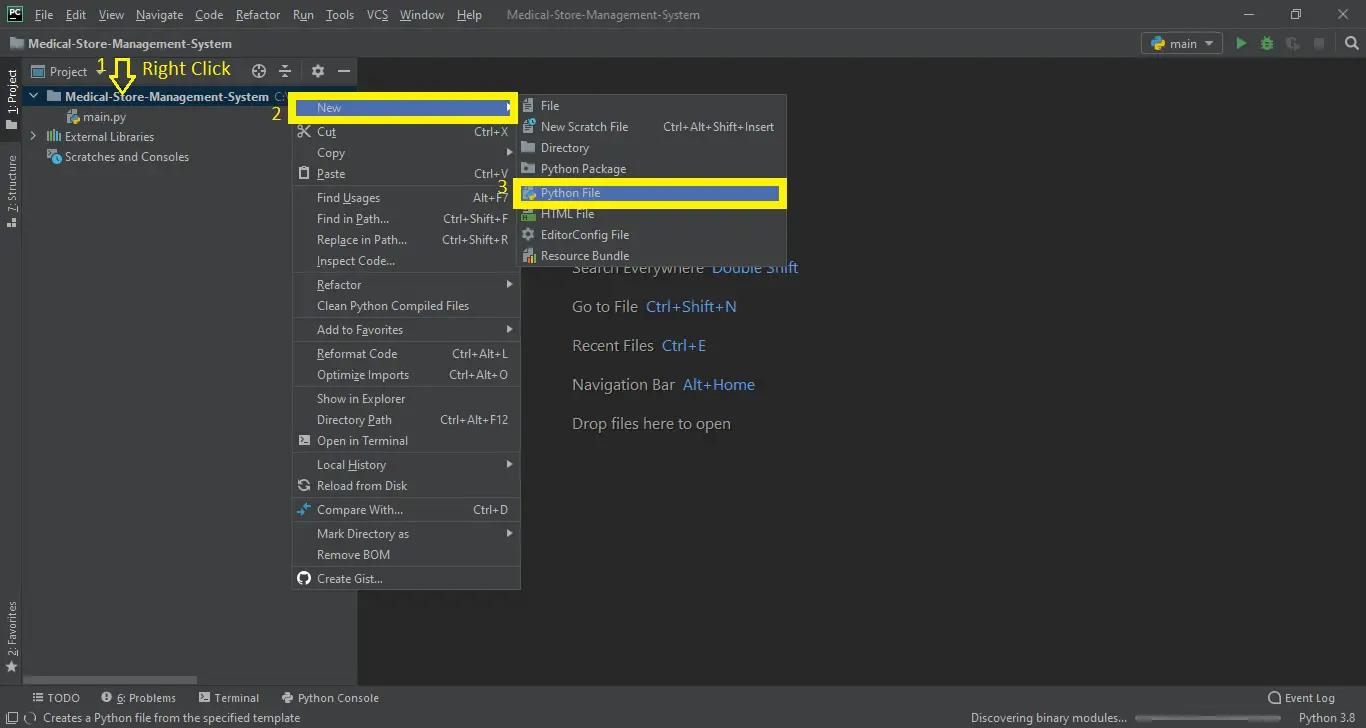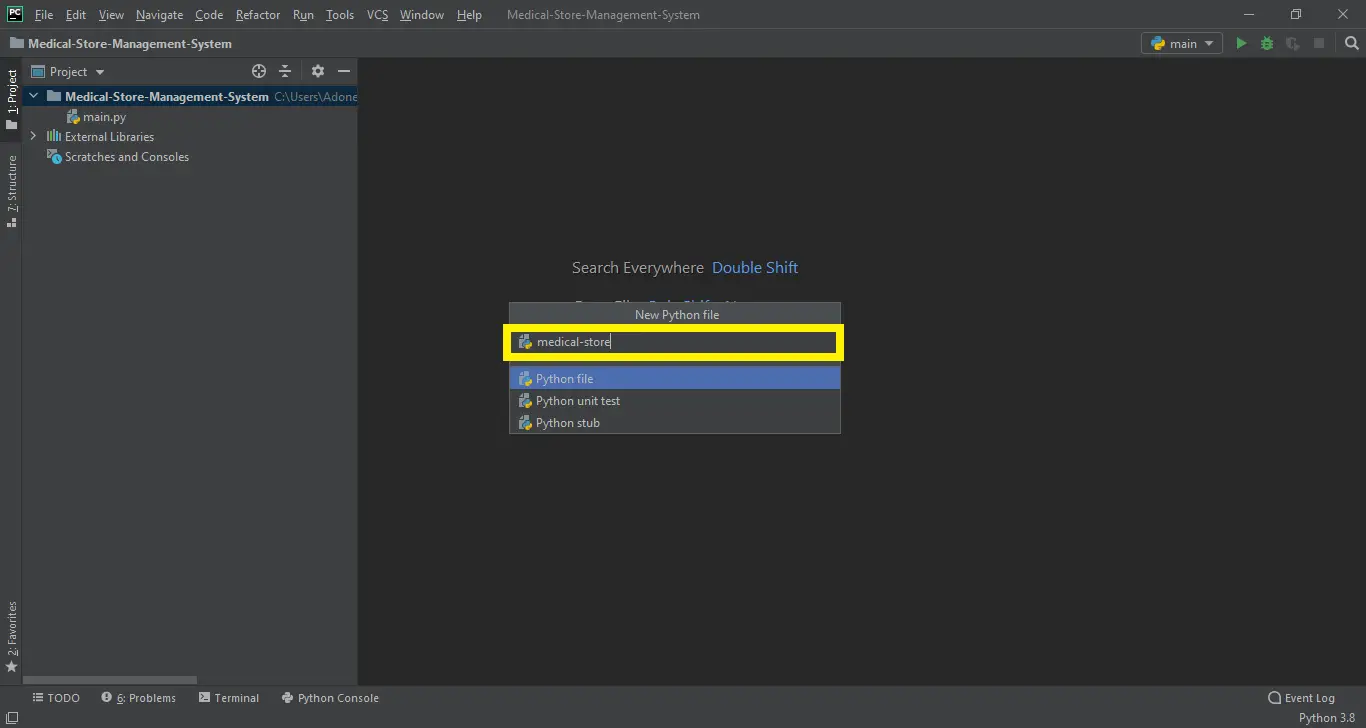# Medical Store Management System Project in Python with Source Code

## Medical Store Management System Project in Python with Source Code

The Medical Store Management System Project in Python is created using python programming language. Medical Store Management System consists of a Python Script (main.py) and a database file. This python projects with source code for Medical Store Management System is primarily based on Tkinter. This system has a graphical user interface applied by using the Python programming language and SQLite3.

This Project System for Medical Store Management has an easy to use interface and is built with respected customer service to allow stock maintenance, database access and electronic documentation for billing purposes. The program also encourages consumer computing discounts, frequent revenue, and identifies measures to avoid possible loss of revenue.

This system platform is best for educational purposes or can be used to simplify the manual maintenance process of records and cash flows by a wide range of retail and wholesale outlets. It’s more versatile and saves you time and money. The Medical Store Management System measures and shows the overall daily sales and also tells the items about the expiry of the coming week.

Medical Store Management System pro...
Medical Store Management System project In Python With Source Code 2021 Free Download

In this tutorial, I will educate you on how to establish Medical Store System, this tutorial consists of the downloadable Medical Store Management System Project in Python where in you alter it to fit your requirements.

Before you start to create a Medical Store Management System Project in Python, make sure you have PyCharm IDE installed in your computer.

By the way if you are new to python programming and you don’t know what would be the the Python IDE to use, I have here a list of Best Python IDE for Windows, Linux, Mac OS that will suit for you. I also have here How to Download and Install Latest Version of Python on Windows.

## Medical Store Management System Project in Python Steps on How to Create a Project

Time needed: 5 minutes.

Here’s the Steps on How to Create a Medical Store Management System Project in Python with Source Code

• Step 1: Create a Project Name.

First, open the PyCharm IDE and click “File” and select “New Project” and then create a project name after that click the “create” button.• Step 2: Create a Python File.

Next, after creating a project name, “right” click the project name and the click “New” after that choose “Python File“.• Step 3: Name the Python File.

Last, choose Python File name the file “Medical-Store-Management-System” and then click “Enter“.• Step 4: The actual code

Finally, you can start coding, you are free to copy the code that being provided below.

## Codefor the Admin main module in Medical Store Management System Project in Python .

In this module displayed the Admin main module of the system that can control all the module of the system.

``````def open_win(): #OPENS MAIN MENU----------------------------------------------------------------------------MAIN MENU
global apt, flag
flag='apt'
apt=Tk()
apt.title("Interface")
Label(apt, text="EVANZ MEDICAL STORE COMPANY").grid(row=0,column=0,columnspan=3)
Label(apt, text='*'*80).grid(row=1,column=0,columnspan=3)
Label(apt, text='-'*80).grid(row=3,column=0,columnspan=3)

Label(apt, text="Stock Maintenance",  bg='green', fg='white').grid(row=2,column=0)
Button(apt,text='New V.C.', width=25, bg='green', fg='white', command=val_cus).grid(row=4,column=0)
Button(apt,text='Add product to Stock', bg='green', fg='white', width=25,command=stock).grid(row=5,column=0)
Button(apt,text='Delete product from Stock', bg='red', fg='white', width=25, command=delete_stock).grid(row=6,column=0)

Label(apt, text="Access Database",  bg='blue', fg='white').grid(row=2,column=1)
Button(apt,text='Modify',width=15,  bg='blue', fg='white', command=modify).grid(row=4,column=1)
Button(apt,text='Search', width=15,  bg='blue', fg='white', command=search).grid(row=5,column=1)
Button(apt,text='Expiry Check',  bg='red', fg='white', width=15, command=exp_date).grid(row=6,column=1)

Label(apt, text="Handle Cash Flows", bg='skyblue', fg='black').grid(row=2,column=2)
Button(apt,text="Check Today's Revenue", bg='skyblue', fg='black', width=20,command=show_rev).grid(row=5,column=2)
Button(apt,text='Billing', width=20, bg='skyblue', fg='black', command=billing).grid(row=4,column=2)
Button(apt,text='Logout', bg='red', fg='white', width=20, command=again).grid(row=6, column=2)
apt.mainloop()``````

## Codefor the add customer in Medical Store Management System Project in Python.

In the code given below, which is for the adding new valued customer.

``````def val_cus():  #to enter new valued customer-----------------------------------------------------------NEW VALUED CUSTOMER
global val, flag, dbt, name_vc, add_vc, cur, c, vc_id
apt.destroy()
cur.execute("select * from cus")
flag='val'
val=Tk()
Label(val, bg='blue', fg='white',text="****ENTER VALUED CUSTOMER DETAILS****").grid(row=0,column=0,columnspan=3)
Label(val,text="-"*60).grid(row=1,column=0,columnspan=3)
Label(val,text="Name: ").grid(row=2,column=0)
name_vc=Entry(val)
name_vc.grid(row=2, column=1)
Label(val,text="Value Id: ").grid(row=4,column=0)
vc_id=Entry(val)
vc_id.grid(row=4, column=1)
Button(val,text='Submit',bg='blue', fg='white',command=val_get).grid(row=5, column=1)
Label(val,text='-'*60).grid(row=6,column=0,columnspan=3)
val.mainloop()``````

## Codefor the add product in Medical Store Management System Project in Python.

In the code given below, which is for the adding new product.

`def stock():    #add to stock window------------------------------------------------------------------------ADD TO STOCK    global cur, c, columns, accept, flag, sto, apt    apt.destroy()    flag='sto'    accept=['']*10    sto=Tk()    sto.title('STOCK ENTRY')    Label(sto,text='ENTER NEW PRODUCT DATA TO THE STOCK').grid(row=0,column=0,columnspan=2)    Label(sto,text='-'*50).grid(row=1,column=0,columnspan=2)    for i in range(1,len(columns)):        Label(sto,width=15,text=' '*(14-len(str(columns[i])))+str(columns[i])+':').grid(row=i+2,column=0)        accept[i]=Entry(sto)        accept[i].grid(row=i+2, column=1)    Button(sto,width=15,text='Submit', bg='blue', fg='white',command=submit).grid(row=12,column=1)    Label(sto,text='-'*165).grid(row=13,column=0,columnspan=7)    Button(sto,width=15,text='Reset', bg='red', fg='white',command=reset).grid(row=12,column=0)    Button(sto,width=15,text='Refresh stock',bg='skyblue', fg='black',command=ref).grid(row=12,column=4)    for i in range(1,6):        Label(sto,text=columns[i]).grid(row=14,column=i-1)    Label(sto,text='Exp           Rack   Manufacturer                      ').grid(row=14,column=5)    Button(sto,width=10,text='Main Menu', bg='green', fg='white',command=main_menu).grid(row=12,column=5)    ref()    sto.mainloop()`

## Codefor the delete product in Medical Store Management System Project in Python.

In the code given below, which is for the function of delete product.

``````def delete_stock(): #OPENS DELETE WINDOW----------------------------------------------------DELETES A PARTICULAR STOCK ITEM
global cur, c, flag, lb1, d
apt.destroy()
flag='d'
d=Tk()
d.title("Delete a product from Stock")
Label(d,text='Enter Product to delete:').grid(row=0,column=0)
Label(d,text='',width=30,bg='white').grid(row=0,column=1)
Label(d,text='Product').grid(row=2,column=0)
Label(d,text='Qty.  Exp.dt.     Cost                           ').grid(row=2,column=1)
ren()
b=Button(d,width=20,text='Delete', bg='red', fg='white',command=delt).grid(row=0,column=3)
d.mainloop()``````

## Codefor the checking expire medicine in Medical Store Management System Project in Python.

In the code given below, which is for the function of checking expire medicine.

``````def exp_date(): # expiry window open-----------------------------------------------------------------------------EXPIRY
global exp, s,c, cur, flag, apt, flags
apt.destroy()
flag='exp'
from datetime import date
now=time.localtime()
n=[]
cur.execute("select *from med")
for i in cur:
n.append(i)
c.commit()
exp=Tk()
exp.title('EXPIRY CHECK')
Label(exp,text='Today : '+str(now)+'/'+str(now)+'/'+str(now)).grid(row=0, column=0, columnspan=3)
Label(exp,text='Selling Expired Medicines and Drugs is Illegal').grid(row=1, column=0,columnspan=3)
Label(exp,text='-'*80).grid(row=2, column=0,columnspan=3)
s=Spinbox(exp,values=n)
s.grid(row=3, column=0)
Button(exp,text='Check Expiry date', bg='red', fg='white', command=s_exp).grid(row=3, column=1)
Label(exp,text='-'*80).grid(row=4, column=0,columnspan=3)
if flags=='apt1':
Button(exp,text='Main Menu', bg='green', fg='white', command=main_cus).grid(row=5, column=2)
else:
Button(exp,width=20,text='Check Products expiring', bg='red', fg='white', command=exp_dt).grid(row=5, column=0)
exp.mainloop()``````

## Codefor the Revenue in Medical Store Management System Project in Python.

In the code given below, which is for the function of total revenue.

``````def show_rev(): # opens revenue window-----------------------------------------------------------------------TOTAL REVENUE
global c, cur, flag,rev
apt.destroy()
flag='rev'
rev=Tk()
total=0.0
today=str(time.localtime())+'/'+str(time.localtime())+'/'+str(time.localtime())
Label(rev,text='Today: '+today).grid(row=0,column=0)
cur.execute('select * from bills')
for i in cur:
if i==today:
total+=float(i)
print (total)
Label(rev,width=22,text='Total revenue: PHP '+str(total), bg='blue',fg='white').grid(row=1,column=0)
cx=0
vsb=Scrollbar(orient='vertical')
lb1=Listbox(rev,width=25, yscrollcommand=vsb.set)
vsb.grid(row=2,column=1,sticky=N+S)
lb1.grid(row=2,column=0)
vsb.config( command = lb1.yview )
cur.execute("select * from bills")
for i in cur:
if i==today:
cx+=1
lb1.insert(cx,'Bill No.: '+str(i)+'    : PHP '+str(i))
c.commit()
rev.mainloop()
``````

## Code for the billing in Medical Store Management System Project in Python.

In the code given below, which is for the function of a customer to pay a bill for there purchase of a medicine.

``````def billing(): # to create bills for customer-------------------------------------------------------------BILLING system
global c, cur, apt, flag, t, name, name1, add, st, names, qty, sl, qtys, vc_id, n, namee, lb1
t=0
vc_id=''
names=[]
qty=[]
sl=[]
n=[]
qtys=['']*10
cur.execute("select *from med")
for i in cur:
n.append(i)
c.commit()
if flag=='st':
st.destroy()
else:
apt.destroy()
flag='st'
st=Tk()
st.title('BILLING SYSTEM')
Label(st,text='-'*48+'BILLING SYSTEM'+'-'*49).grid(row=0,column=0,columnspan=7)
Label(st,text='Enter Name: ').grid(row=1,column=0)
name1=Entry(st)
name1.grid(row=1, column=1)
Label(st,text="Value Id (if available)").grid(row=3, column=0)
vc_id=Entry(st)
vc_id.grid(row=3, column=1)
Button(st,text='Check V.C.', bg='green', fg='white', command=blue).grid(row=4, column=0)
Label(st,text='-'*115).grid(row=6, column=0,columnspan=7)
Label(st,text='SELECT PRODUCT',width=25,relief='ridge').grid(row=7, column=0)
Label(st,text=' RACK  QTY LEFT     COST          ',width=25,relief='ridge').grid(row=7, column=1)
Button(st,text='Add to bill', bg='blue', fg='white', width=15,command=append2bill).grid(row=8, column=6)
Label(st,text='QUANTITY',width=20,relief='ridge').grid(row=7, column=5)
qtys=Entry(st)
qtys.grid(row=8,column=5)
refresh()
Button(st,width=15,text='Refresh Stock',bg='skyblue', fg='black', command=refresh).grid(row=3,column=6)
Button(st,width=15,text='Reset Bill', bg='red', fg='white', command=billing).grid(row=4,column=6)
Button(st,width=15,text='Print Bill', bg='orange', fg='white', command=print_bill).grid(row=5,column=6)
Button(st,width=15,text='Save Bill', bg='blue', fg='white', command=make_bill).grid(row=7,column=6)

st.mainloop()``````

Anyway, if you want to level up your programming knowledge, especially python, try this new article I’ve made for you Best Python Projects with source code for Beginners. But If you’re going to focus on web development using Django, you can download here from our list of Best Django Projects with source code based on real-world projects.

## How To Run The Medical Store Management System Project in Python with Source Code?

To run this project, you must have installed a Pycharm on your PC (for Windows). This Medical Store Management System Project in Python with Source Code is free to download, Use for educational purposes only!

1st Step: Unzip the file or Extract the file

2nd Step: Double click the main.py

3rd Step: Project is ready to run